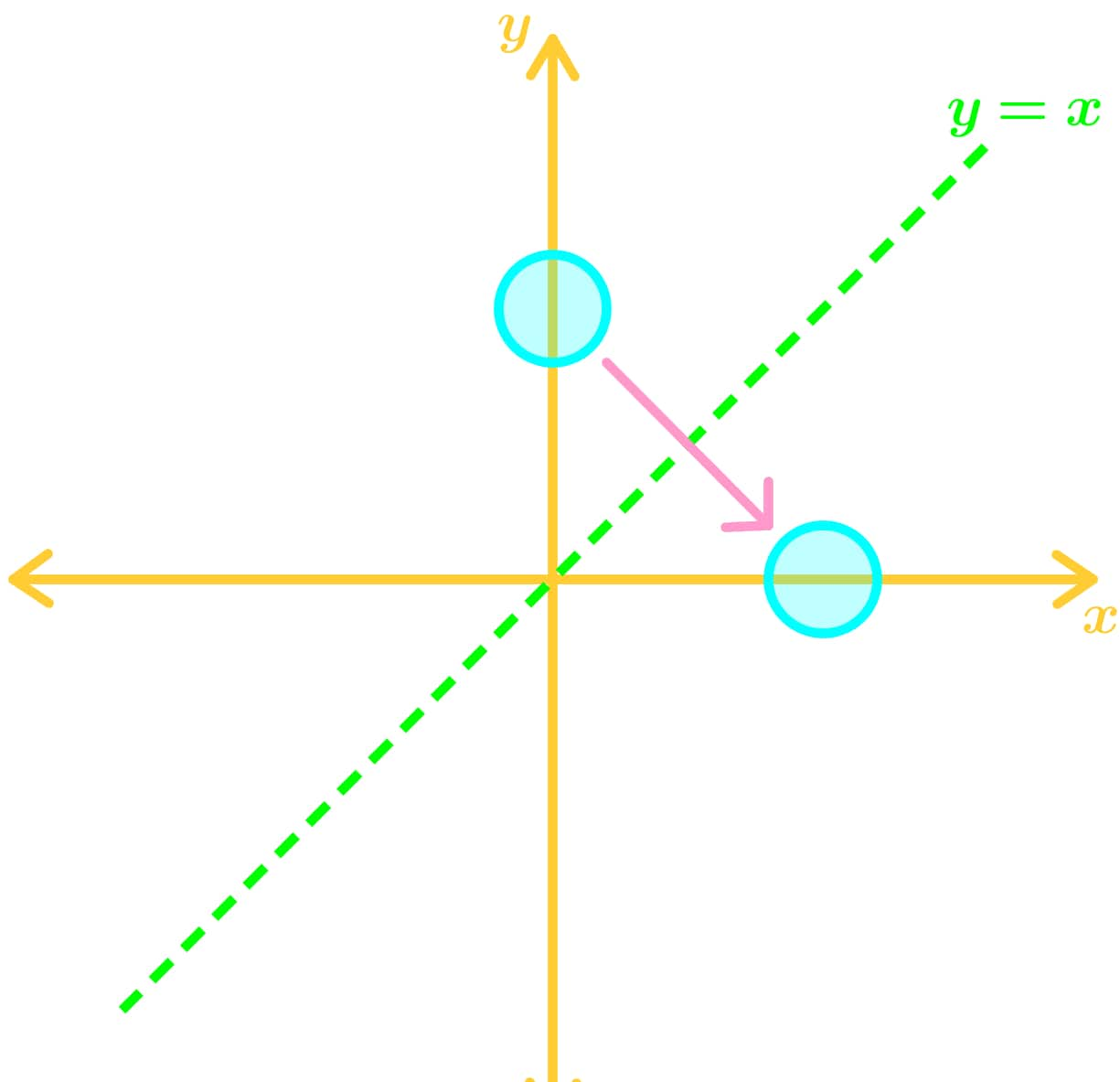# Finding the transformation matrix

### Finding the transformation matrix

We are always given the transformation matrix to transform shapes and vectors, but how do we actually give the transformation matrix in the first place? To do this, we must take a look at two unit vectors. With each unit vector, we will imagine how they will be transformed. Then take the two transformed vector, and merged them into a matrix. That matrix will be the transformation matrix. We will first examine the different types of transformations we will encounter, and then learn how to find the transformation matrix when given a graph.

#### Lessons

In this section, we will be focusing on finding the transformation matrix.
Given a picture or a description of the transformation, how do we find the transformation matrix? What we do is to take a look at the two unit vectors: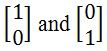We want to ask ourselves how the transformation given in the question changes these two unit vectors.
Let's say that the unit vector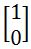transforms fromto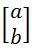and the unit vector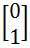transforms fromto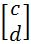. Then we say that: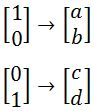Then we combine these two column vectors into one matrix.
Hence, the transformation matrix is: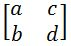• Introduction
Finding the transformation matrix overview

• 1.
Transformation of vectors
You are given a vector and a description of the transformation. Determine the new vector when it is transformed, and graph them:
a)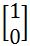, scaled by a factor of 4

b)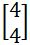, scaled by a factor of $\frac{1}{2}$

c)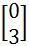, rotated 90° counter-clockwise

d)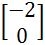, rotated 270° clockwise

e)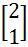, reflected on the $x$-axis

f)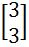, reflected on the $y$-axis

• 2.
Finding the transformation matrix
You are given a picture of a transformation taking place. Find the matrix that causes this transformation:
a)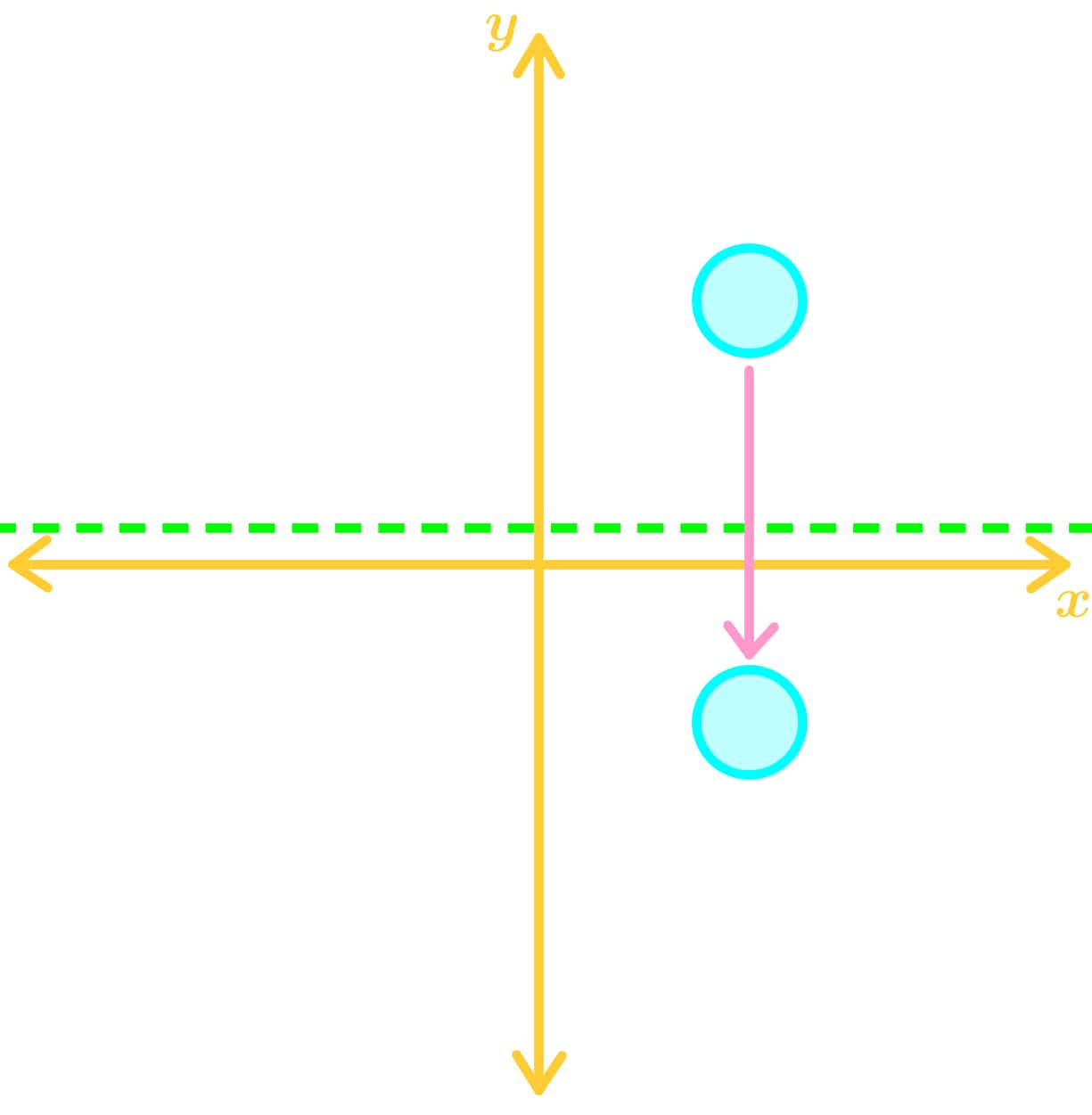b)
scaled by a factor of $\frac{1}{3}$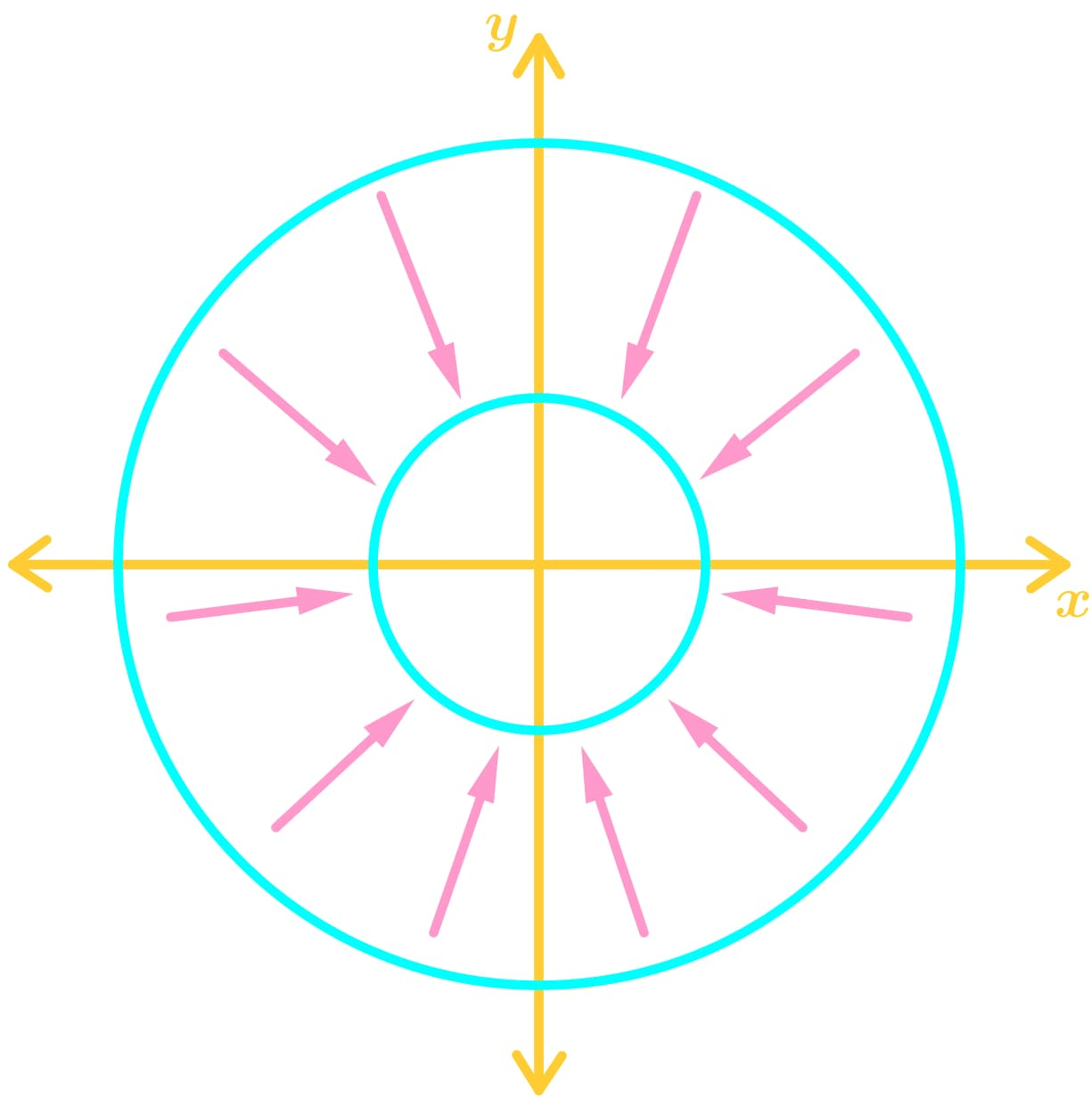c)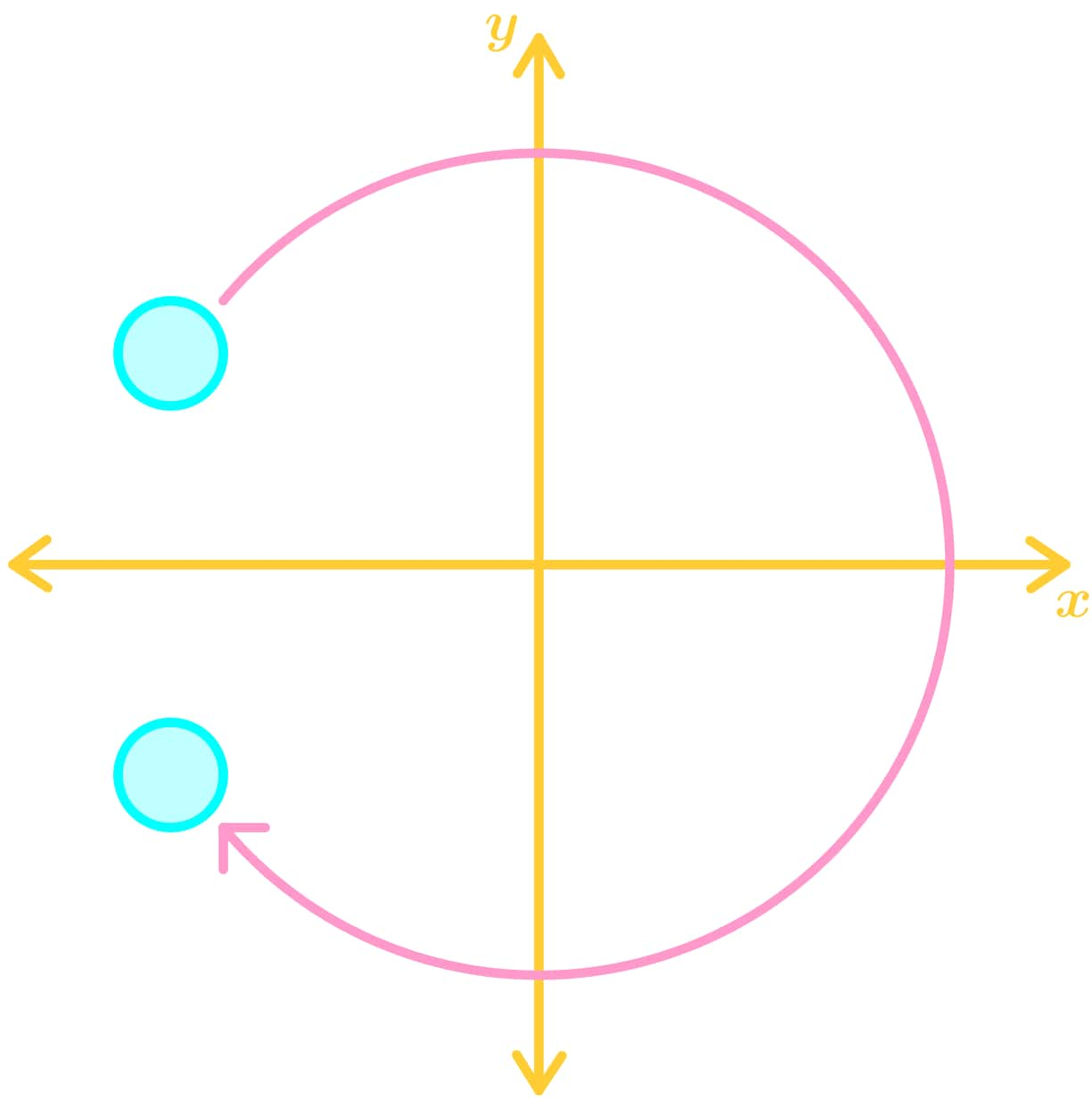d)